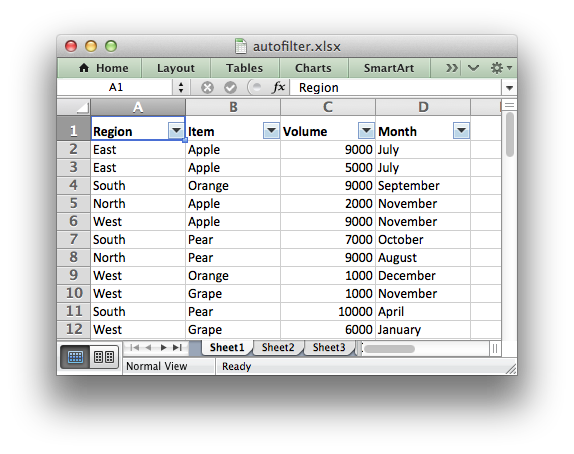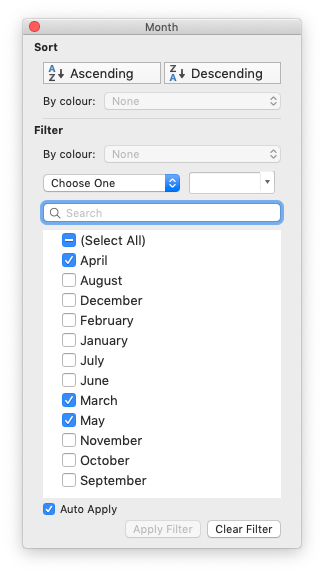# Working with Autofilters

An autofilter in Excel is a way of filtering a 2D range of data based on some simple criteria.## Applying an autofilter

The first step is to apply an autofilter to a cell range in a worksheet using the `autofilter()` method:

```worksheet.autofilter('A1:D11')
```

As usual you can also use Row-Column notation:

```worksheet.autofilter(0, 0, 10, 3)  # Same as above.
```

## Filter data in an autofilter

The `autofilter()` defines the cell range that the filter applies to and creates drop-down selectors in the heading row. In order to filter out data it is necessary to apply some criteria to the columns using either the `filter_column()` or `filter_column_list()` methods.

The `filter_column` method is used to filter columns in a autofilter range based on simple criteria:

```worksheet.filter_column('A', 'x > 2000')
worksheet.filter_column('B', 'x > 2000 and x < 5000')
```

It isn’t sufficient to just specify the filter condition. You must also hide any rows that don’t match the filter condition. Rows are hidden using the `set_row()` `hidden` parameter. `XlsxWriter` cannot filter rows automatically since this isn’t part of the file format.

The following is an example of how you might filter a data range to match an autofilter criteria:

```# Set the autofilter.
worksheet.autofilter('A1:D51')

# Add the filter criteria. The placeholder "Region" in the filter is
# ignored and can be any string that adds clarity to the expression.
worksheet.filter_column(0, 'Region == East')

# Hide the rows that don't match the filter criteria.
row = 1
for row_data in (data):
region = row_data

# Check for rows that match the filter.
if region == 'East':
# Row matches the filter, display the row as normal.
pass
else:
# We need to hide rows that don't match the filter.
worksheet.set_row(row, options={'hidden': True})

worksheet.write_row(row, 0, row_data)

# Move on to the next worksheet row.
row += 1
```

## Setting a filter criteria for a column

The `filter_column()` method can be used to filter columns in a autofilter range based on simple conditions:

```worksheet.filter_column('A', 'x > 2000')
```

The `column` parameter can either be a zero indexed column number or a string column name.

The following operators are available for setting the filter criteria:

```Operator
==
!=
>
<
>=
<=

and
or
```

An expression can comprise a single statement or two statements separated by the `and` and `or` operators. For example:

```'x <  2000'
'x >  2000'
'x == 2000'
'x >  2000 and x <  5000'
'x == 2000 or  x == 5000'
```

Filtering of blank or non-blank data can be achieved by using a value of `Blanks` or `NonBlanks` in the expression:

```'x == Blanks'
'x == NonBlanks'
```

Excel also allows some simple string matching operations:

```'x == b*'      # begins with b
'x != b*'      # doesn't begin with b
'x == *b'      # ends with b
'x != *b'      # doesn't end with b
'x == *b*'     # contains b
'x != *b*'     # doesn't contain b
```

You can also use `'*'` to match any character or number and `'?'` to match any single character or number. No other regular expression quantifier is supported by Excel’s filters. Excel’s regular expression characters can be escaped using `'~'`.

The placeholder variable `x` in the above examples can be replaced by any simple string. The actual placeholder name is ignored internally so the following are all equivalent:

```'x     < 2000'
'col   < 2000'
'Price < 2000'
```

A filter condition can only be applied to a column in a range specified by the `autofilter()` method.

## Setting a column list filter

Prior to Excel 2007 it was only possible to have either 1 or 2 filter conditions such as the ones shown above in the `filter_column()` method.

Excel 2007 introduced a new list style filter where it is possible to specify 1 or more ‘or’ style criteria. For example if your column contained data for the months of the year you could filter the data based on certain months:The `filter_column_list()` method can be used to represent these types of filters:

```worksheet.filter_column_list('A', ['March', 'April', 'May'])
```

One or more criteria can be selected:

```worksheet.filter_column_list('A', ['March'])
worksheet.filter_column_list('B', [100, 110, 120, 130])
```

To filter blanks as part of the list use Blanks as a list item:

```worksheet.filter_column_list('A', ['March', 'April', 'May', 'Blanks'])
```

As explained above, it isn’t sufficient to just specify filters. You must also hide any rows that don’t match the filter condition.

## Example

See Example: Applying Autofilters for a full example of all these features.